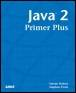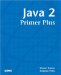# Complex Class MethodsJava™ 2 Primer PlusBy Steven Haines, Steve Potts Table of Contents Chapter 5.  Methods

Your classes are not limited to defining a single method, on the contrary you will define multiple methods in most of your classes. Consider a more complete mathematics class, you might want to have methods not only to square a number, but also cube a number or raise a number to any particular power. Furthermore, you might want to add in your prime number checker, a factorial method, or a square root method.

Listing 5.2 defines a class with a more complex and complete set of methods.

##### Listing 5.2 Math2.java
` 1:  public class Math2 {  2:    public static int square( int n ) {  3:      return n*n;  4:    }  5:  6:    public static int cube( int n ) {  7:      return n*n*n;  8:    }  9:  10:   public static int toThePower( int n, int power ) {  11:     int result=n;  12:     for( int i=0; i<power; i++ ) {  13:        result *= n;  14:     }  15:     return result;  16:   }  17:  18:   public static int factorial( int n ) {  19:     int result = n;  20:     for( int i=n-1; i>0; i-- ) {  21:        result *= i;  22:     }  23:     return result;  24:   }  25:  26:   public static boolean isPrime( int n ) {  27:     // This will left as one of your exercises  28:     return false;  29:   }  30:  31:   public static void showNumber( String operation, int n ) {  32:     System.out.println( "The result of the " + operation + " is " + n );   33:   }  34:  35:   public static void main( String[] args ) {  36:     int a = 5;  37:     showNumber( "Square", square( a ) );  38:     showNumber( "Cube", cube( a ) );  39:     showNumber( "To The Power", toThePower( a, 4 ) );  40:     showNumber( "Factorial", factorial( a ) );  41:   }  42: } `

Listing 5.2 defines six methods:

• square

• cube

• toThePower

• factorial

• isPrime

• showNumber

The first five methods are mathematical and the last is a convenience method so that you don't have to manually type the output each time. square, cube, and toThePower should be self-explanatory; note however that toThePower has a parameter list with two values: the number to raise to the specified power (n), and the power to raise that number to (power).

In mathematics there is the concept of factorial, denoted in mathematical notation (but not Java notation) by an exclamation point (!), which is a number multiplied by every integer number from 1 to the number itself. In this example, the 5 factorial is

` 5! = 5*4*3*2*1 = 120 `

The factorial method in Listing 5.2 shows an iterative approach to solving this problem.

The isPrime method, because it is an exercise question, is left for you to do at the end of this chapter.

The main method defines an int variable named a, assigns it the value 5, and then calls each of the mathematical methods on it, passing the result to showNumber for output.TopJava 2 Primer Plus
ISBN: 0672324156
EAN: 2147483647
Year: 2001
Pages: 332

Similar book on Amazon